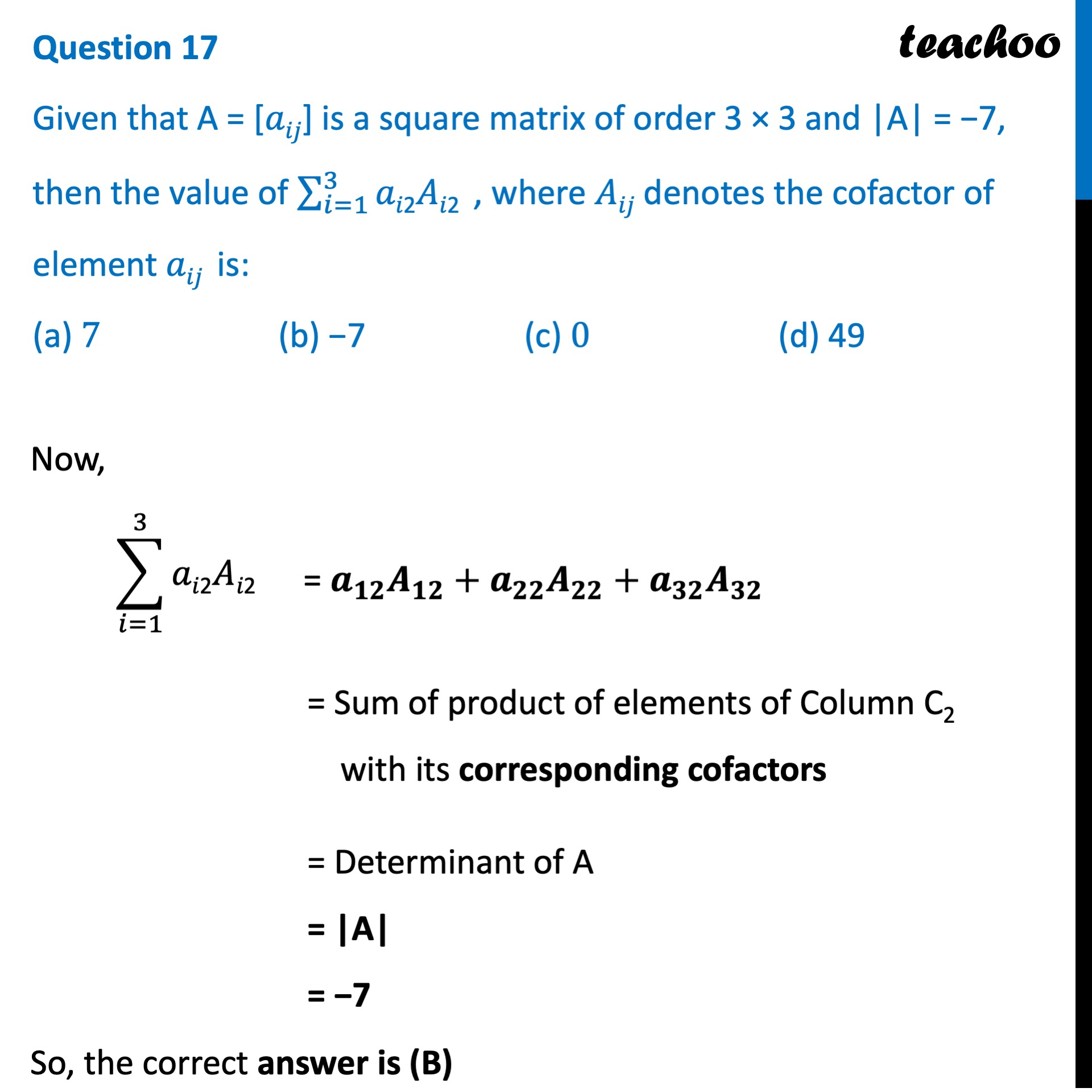CBSE Class 12 Sample Paper for 2022 Boards (MCQ Based - for Term 1)

Class 12
Solutions of Sample Papers and Past Year Papers - for Class 12 Boards

## (a) 7  (b) −7  (c) 0  (d) 49Learn in your speed, with individual attention - Teachoo Maths 1-on-1 Class

### Transcript

Question 17 Given that A = [𝑎𝑖𝑗] is a square matrix of order 3 × 3 and |A| = −7, then the value of ∑_(𝑖=1)^3▒"𝑎𝑖2𝐴𝑖2 " , where 𝐴𝑖𝑗 denotes the cofactor of element 𝑎𝑖𝑗 is: (a) 7 (b) −7 (c) 0 (d) 49 Now, = 𝒂_𝟏𝟐 𝑨_𝟏𝟐+𝒂_𝟐𝟐 𝑨_𝟐𝟐+𝒂_𝟐𝟐 𝑨_𝟏𝟐 = Sum of product of elements of Row R2 with its corresponding cofactors = Determinant of A = |A| = 7 So, the correct answer is (B)